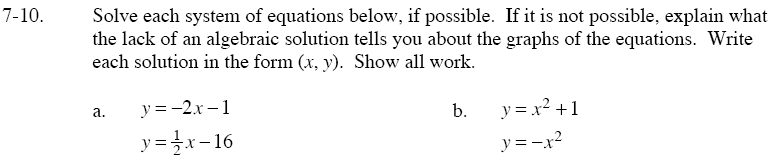### Home > GC > Chapter 7 > Lesson 7.1.1 > Problem7-10

7-10.By the Equal Values Method,

$-2x-1=\frac{1}{2}x-16$

x = 6

Substitute 6 for x in one of the original equations.
Solve for y, which will give you the intersection of the two lines.

By the Equal Values Method,

$x^2+1=-\textit{x}^2$

$2\textit{x}^2+1=0$

$x=\frac{0\pm\sqrt{0^2=(4)(2)(1)}}{2\cdot2}\;\to\;x=\frac{0\pm\sqrt{-8}}{4}$